# Chapter 04 What The Probability That You Will ReceiveType Homework HelpPages 9Words 4165Authors J.K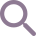### Unlock document.

This document is partially blurred.
Unlock all pages and 1 million more documents.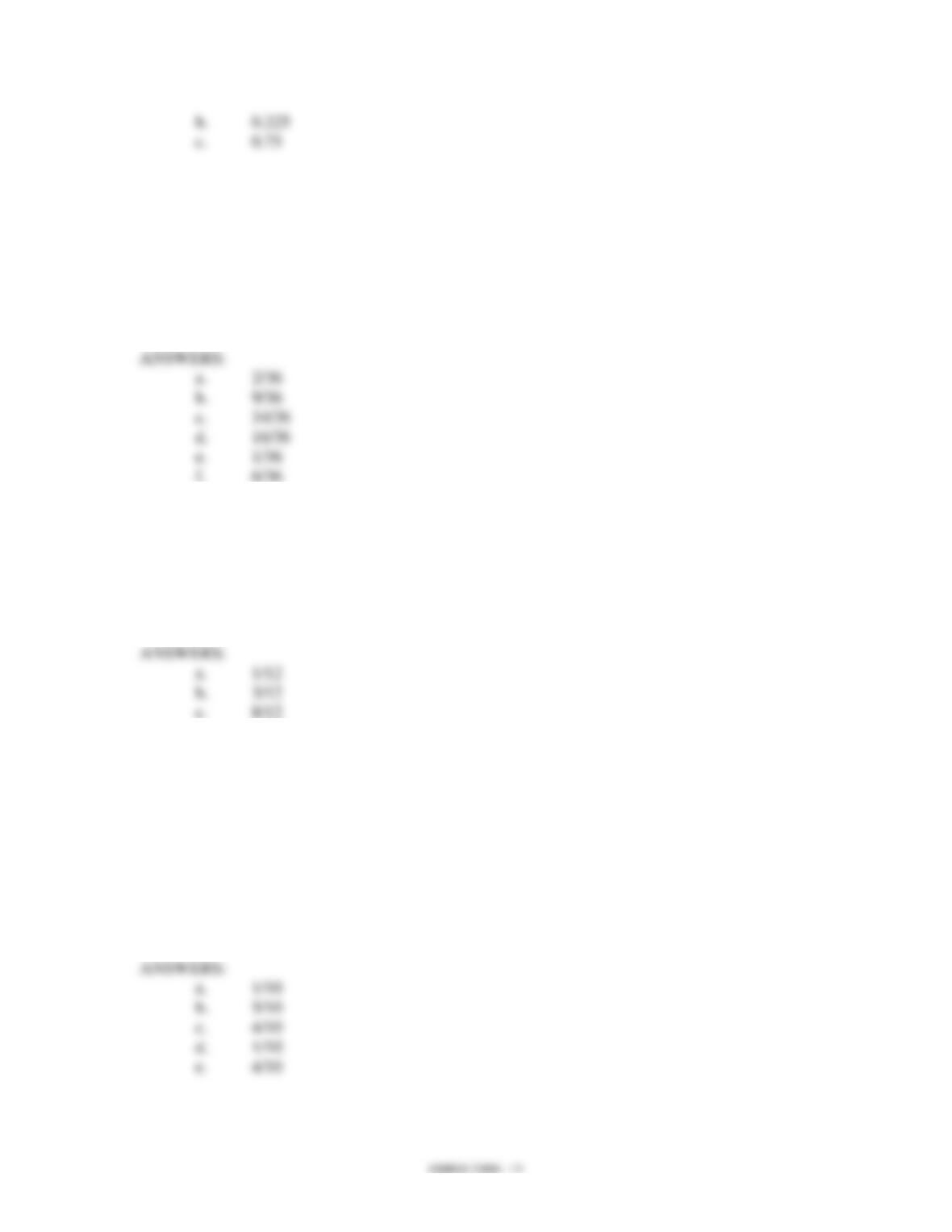7. An experiment consists of throwing two six-sided dice and observing the number of spots
on the upper faces. Determine the probability that
a. the sum of the spots is 3.
b. each die shows four or more spots.
c. the sum of the spots is not 3.
d. neither a one nor a six appear on each die.
e. a pair of sixes appear.
f. the sum of the spots is 7.
8. Assume that in your hand you hold an ordinary six-sided die and a dime. You toss both
the die and the dime on a table.
a. What is the probability that a head appears on the dime and a six on the die?
b. What is the probability that a tail appears on the dime and any number more than
3 on the die?
c. What is the probability that a number larger than 2 appears on the die?
9. A very short quiz has one multiple-choice question with five possible choices (a, b, c, d,
e) and one true or false question. Assume you are taking the quiz but do not have any
idea what the correct answer is to either question, but you mark an answer anyway.
a. What is the probability that you have given the correct answer to both questions?
b. What is the probability that only one of the two answers is correct?
c. What is the probability that neither answer is correct?
d. What is the probability that only your answer to the multiple-choice question is
correct?
e. What is the probability that you have only answered the true or false question
correctly?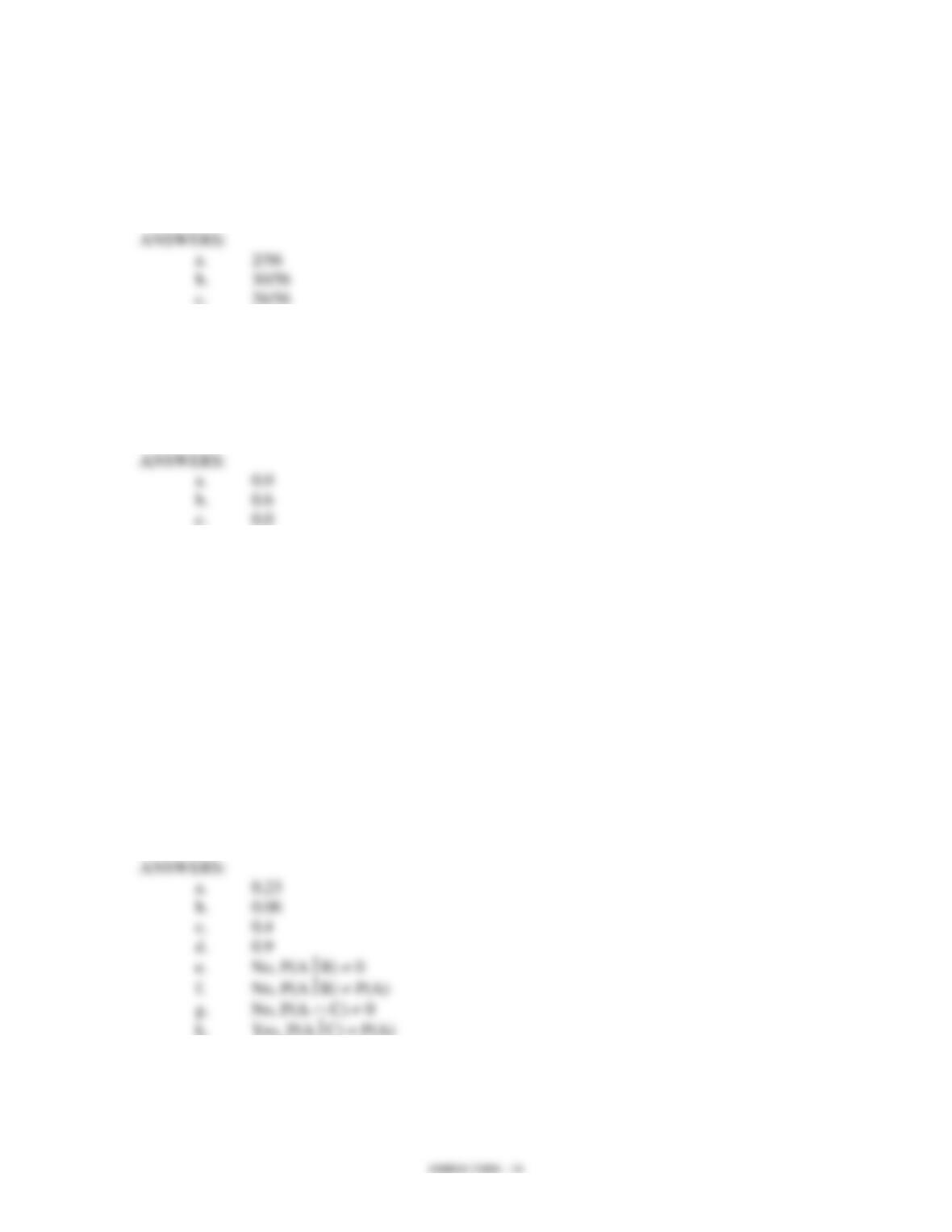10. Two of the cylinders in an eight-cylinder car are defective and need to be replaced. If two
cylinders are selected at random, what is the probability that
a. both defective cylinders are selected?
b. no defective cylinder is selected?
c. at least one defective cylinder is selected?
11. Assume two events A and B are mutually exclusive and, furthermore, P(A) = 0.2 and
P(B) = 0.4.
a. Find P(A B).
b. Find P(A B).
c. Find P(AB).
12. You are given the following information on Events A, B, C, and D.
P(A) = .4
P(A D) = .6
P(A C) = .04
P(B) = .2
P(AB) = .3
P(A D) = .03
P(C) = .1
a. Compute P(D).
b. Compute P(A B).
c. Compute P(AC).
d. Compute the probability of the complement of C.
13. A government agency has 6,000 employees. The employees were asked whether they
preferred a four-day work week (10 hours per day), a five-day work week (8 hours per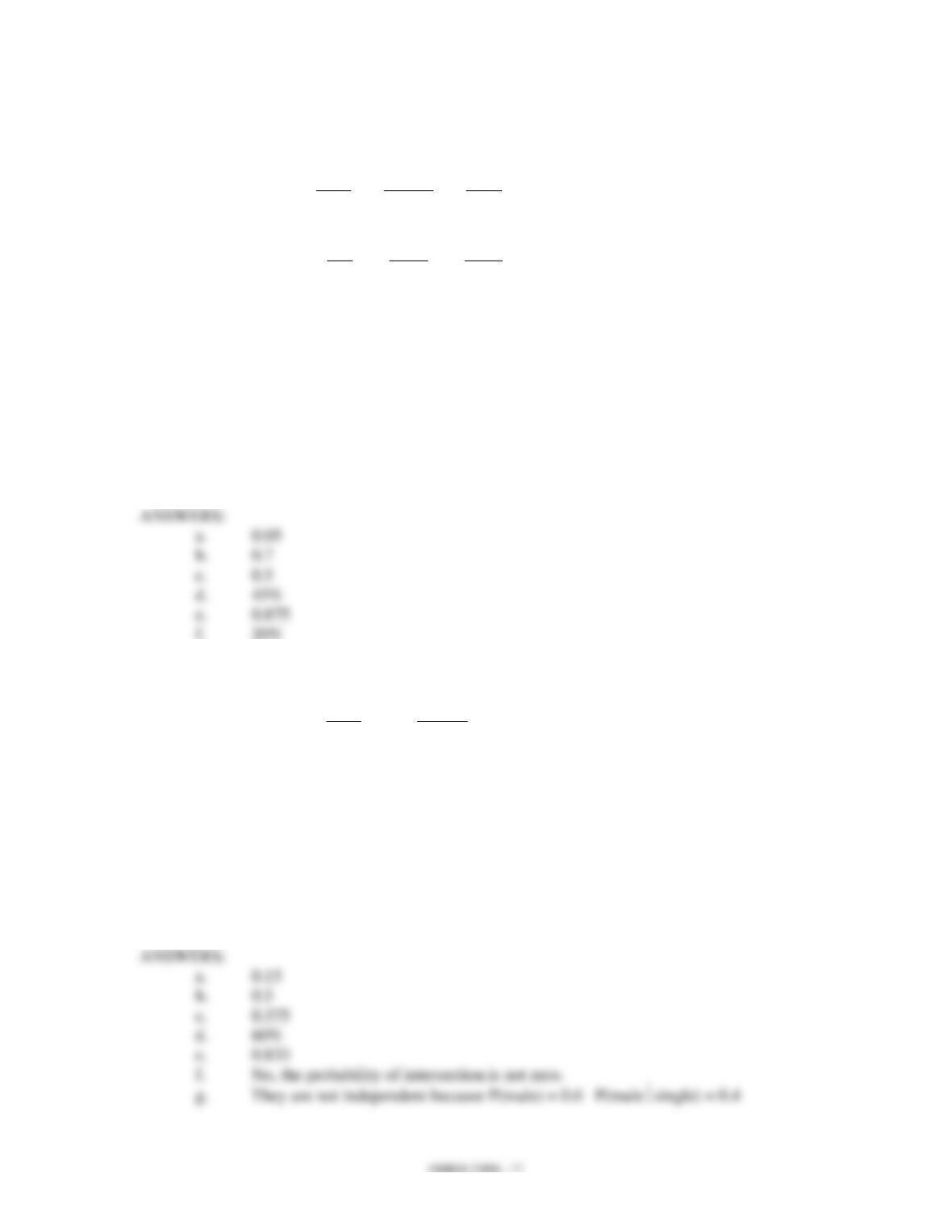day), or flexible hours. You are given information on the employees’ responses broken
down by gender.
Male
Female
Total
Four days
300
600
900
Five days
1,200
1,500
2,700
Flexible
300
2,100
2,400
Total
1,800
4,200
6,000
a. What is the probability that a randomly selected employee is a man and is in
favor of a four-day work week?
b. What is the probability that a randomly selected employee is female?
c. A randomly selected employee turns out to be female. Compute the probability
that she is in favor of flexible hours.
d. What percentage of employees is in favor of a five-day work week?
e. Given that a person is in favor of flexible time, what is the probability that the
person is female?
f. What percentage of employees is male and in favor of a five-day work week?
14. A bank has the following data on the gender and marital status of 200 customers.
Male
Single
20
Married
100
a. What is the probability of finding a single female customer?
b. What is the probability of finding a married male customer?
c. If a customer is female, what is the probability that she is single?
d. What percentage of customers is male?
e. If a customer is male, what is the probability that he is married?
f. Are gender and marital status mutually exclusive?
g. Is marital status independent of gender? Explain using probabilities.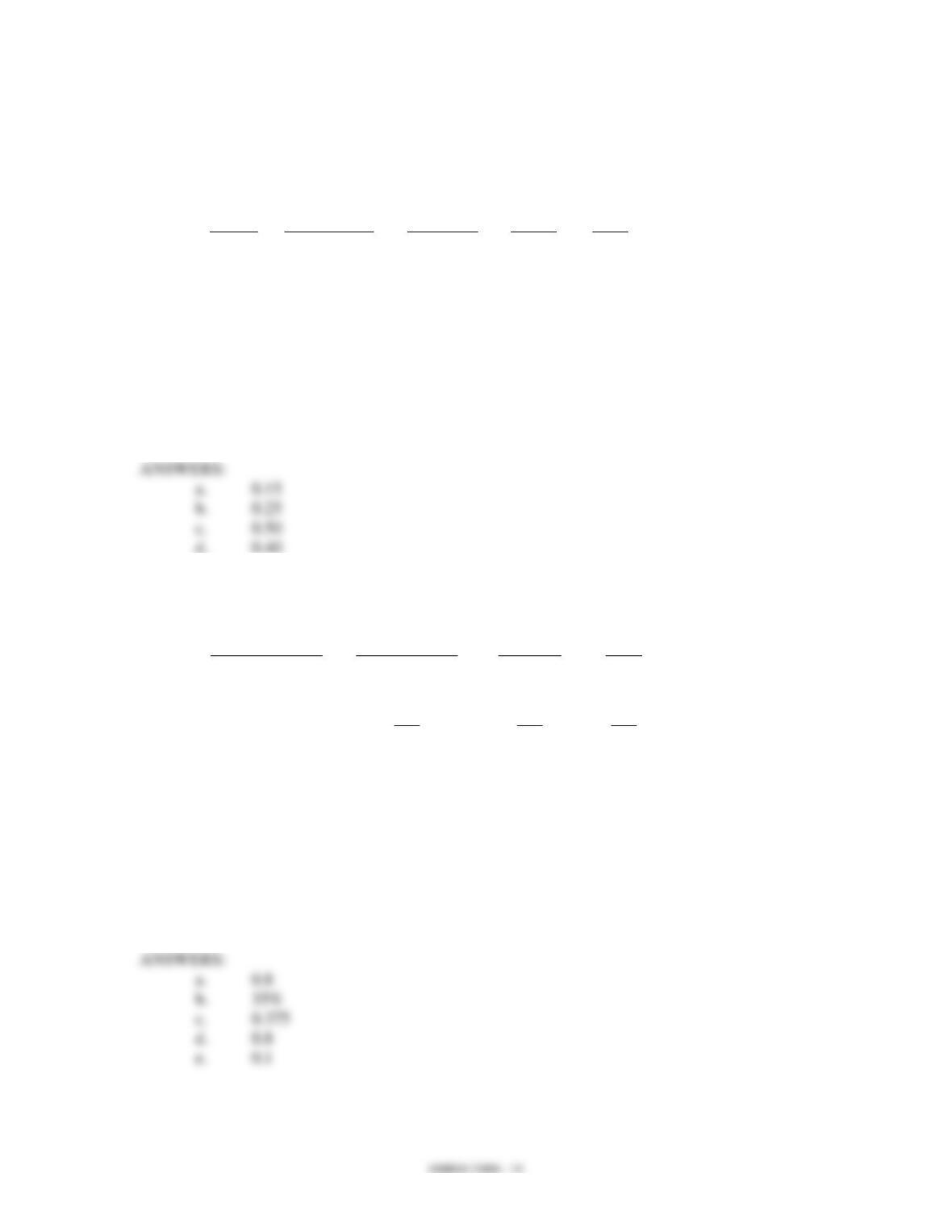15. A survey of a sample of business students resulted in the following information regarding
the genders of the individuals and their major.
Major
Gender
Management
Marketing
Others
Total
Male
40
10
30
80
Female
30
20
70
120
Total
70
30
100
200
a. What is the probability of selecting an individual who is majoring in Marketing?
b. What is the probability of selecting an individual who is majoring in
Management, given that the person is female?
c. Given that a person is male, what is the probability that he is majoring in
Management?
d. What is the probability of selecting a male individual?
16. The following table shows the number of students in three different degree programs and
Degree Program
Total
150
50
200
Engineering
150
25
175
Arts & Sciences
100
25
125
Total
400
100
500
a. What is the probability that a randomly selected student is an undergraduate?
b. What percentage of students is engineering majors?
c. If we know that a selected student is an undergraduate, what is the probability
that he or she is a business major?
d. A student is enrolled in the Arts and Sciences school. What is the probability
that the student is an undergraduate student?
e. What is the probability that a randomly selected student is a graduate Business
major?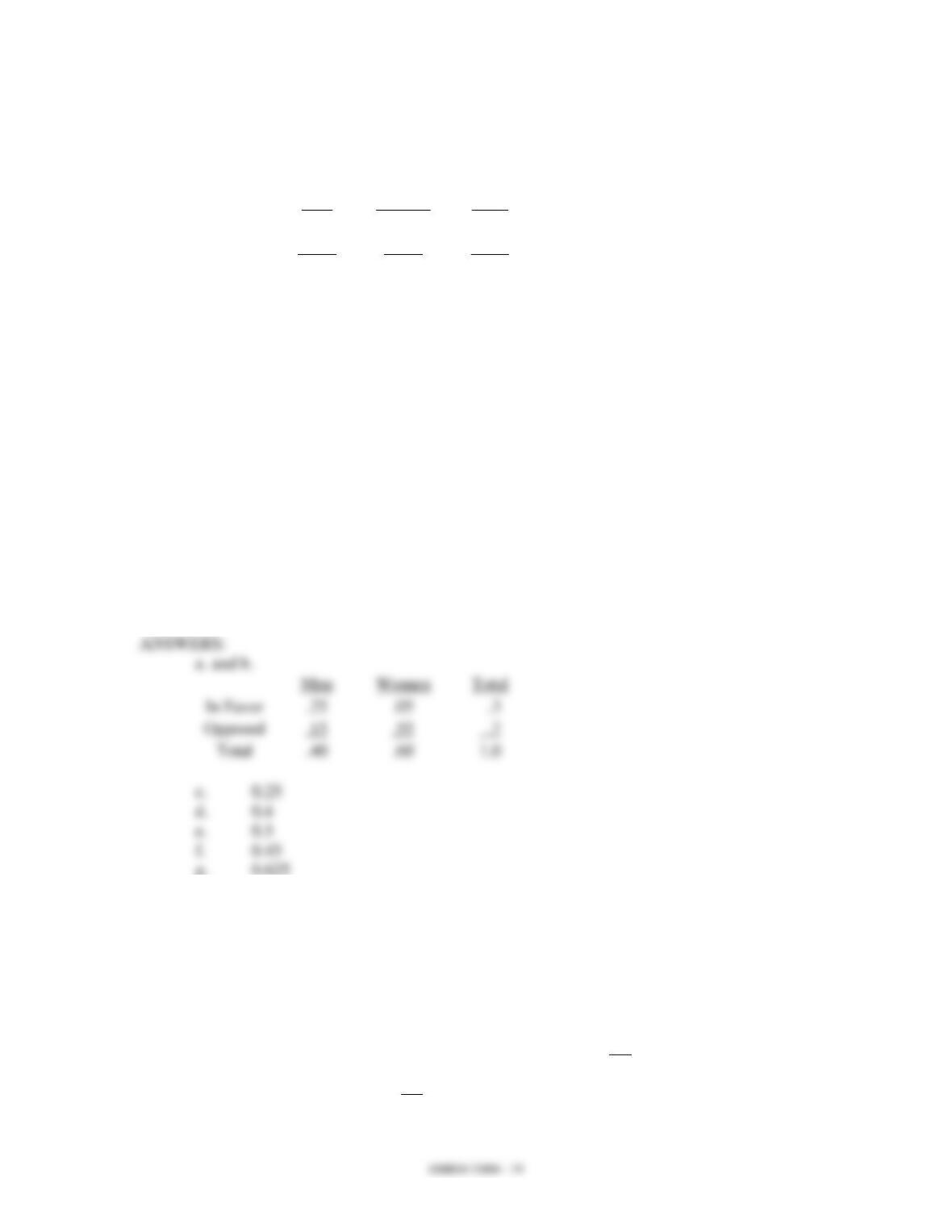17. A small town has 5,600 residents. The residents in the town were asked whether or not
they favored building a new bridge across the river. You are given the following
information on the residents' responses, broken down by gender.
Men
Women
Total
In Favor
1,400
280
1,680
Opposed
840
3,080
3,920
Total
2,240
3,360
5,600
Let: M be the event a resident is a man
W be the event a resident is a woman
F be the event a resident is in favor
P be the event a resident is opposed
a. Find the joint probability table.
b. Find the marginal probabilities.
c. What is the probability that a randomly selected resident is a man and is in favor
of building the bridge?
d. What is the probability that a randomly selected resident is a man?
e. What is the probability that a randomly selected resident is in favor of building
the bridge?
f. What is the probability that a randomly selected resident is a man or in favor of
building the bridge or both?
g. A randomly selected resident turns out to be male. Compute the probability that
he is in favor of building the bridge.
18. On a recent holiday evening, a sample of 500 drivers was stopped by the police. Three
hundred were under 30 years of age. A total of 250 were under the influence of alcohol.
Of the drivers under 30 years of age, 200 were under the influence of alcohol.
Let A be the event that a driver is under the influence of alcohol.
Let Y be the event that a driver is less than 30 years old.
a. Determine P(A) and P(Y).
b. What is the probability that a driver is under 30 and not under the influence of
alcohol?
c. Given that a driver is not under 30, what is the probability that he/she is under the
influence of alcohol?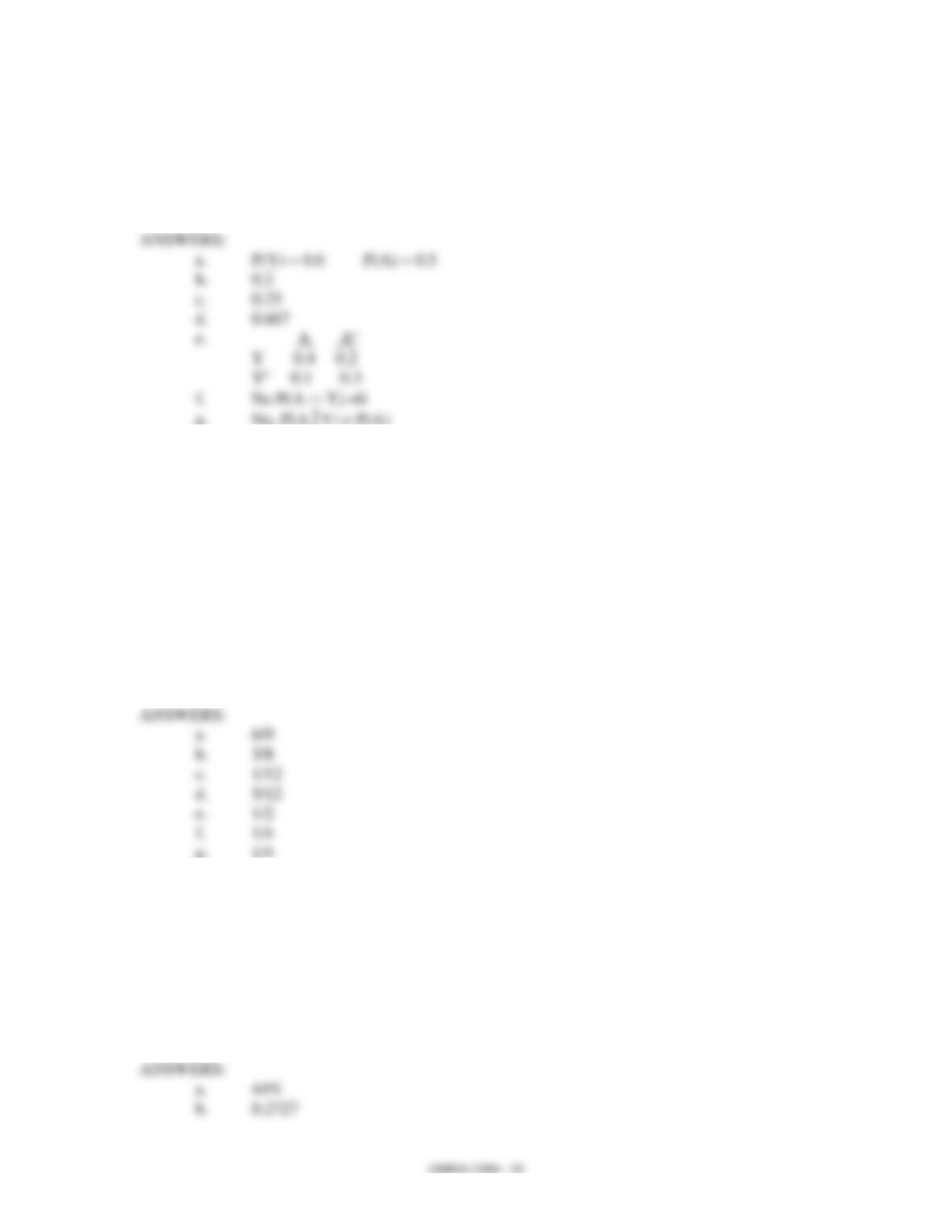d. What is the probability that a driver is under the influence of alcohol if we know
the driver is under 30?
e. Show the joint probability table.
f. Are A and Y mutually exclusive events? Explain.
g. Are A and Y independent events? Explain.
19. Six vitamin and three sugar tablets identical in appearance are in a box. One tablet is
taken at random and given to Person A. A tablet is then selected and given to Person B.
What is the probability that
a. Person A was given a vitamin tablet?
b. Person B was given a sugar tablet given that Person A was given a vitamin
tablet?
c. neither was given vitamin tablets?
d. both were given vitamin tablets?
e. exactly one person was given a vitamin tablet?
f. Person A was given a sugar tablet and Person B was given a vitamin tablet?
g. Person A was given a vitamin tablet and Person B was given a sugar tablet?
20. In a random sample of UTC students 50% indicated they are business majors, 40%
engineering majors, and 10% other majors. Of the business majors, 60% were females;
whereas, 30% of engineering majors were females. Finally, 20% of the other majors
were female.
a. What percentage of students in this sample was female?
b. Given that a person is female, what is the probability that she is an engineering
major?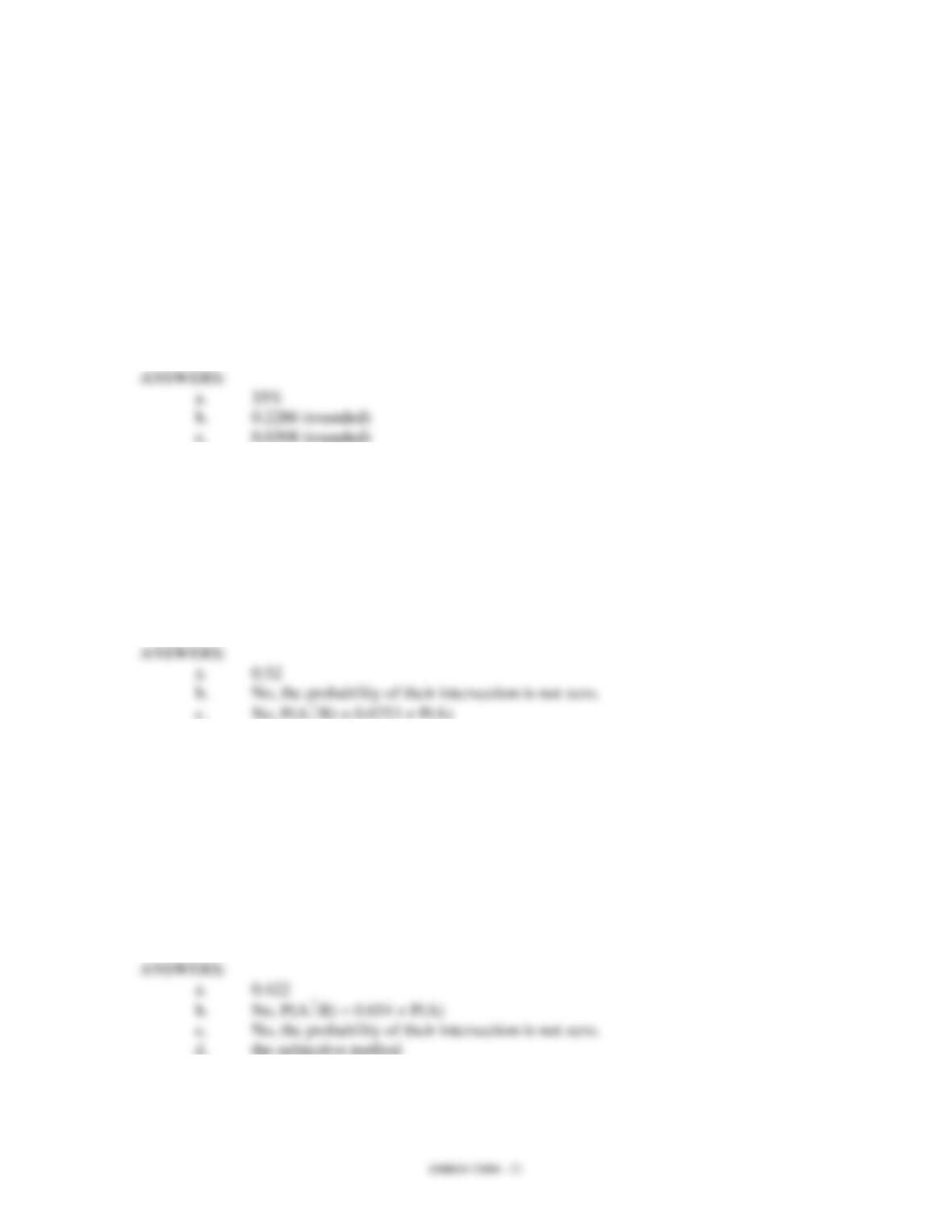21. Sixty percent of the student body at UTC is from the state of Tennessee (T), 30% percent
are from other states (O), and the remainder is international students (I). Twenty percent
of students from Tennessee live in the dormitories, whereas 50% of students from other
states live in the dormitories. Finally, 80% of the international students live in the
dormitories.
a. What percentage of UTC students lives in the dormitories?
b. Given that a student lives in the dormitory, what is the probability that she/he is
an international student?
c. Given that a student does not live in the dormitory, what is the probability that
she/he is an international student?
22. Tammy is a general contractor and has submitted two bids for two projects (A and B).
The probability of getting project A is 0.65. The probability of getting project B is 0.77.
The probability of getting at least one of the projects is 0.90.
a. What is the probability that she will get both projects?
b. Are the events of getting the two projects mutually exclusive? Explain, using
probabilities.
c. Are the two events independent? Explain, using probabilities.
23. Assume you are taking two courses this semester (A and B). Based on your opinion, you
believe the probability that you will pass course A is 0.835; the probability that you will
pass both courses is 0.276. You further believe the probability that you will pass at least
one of the courses is 0.981.
a. What is the probability that you will pass course B?
b. Is the passing of the two courses independent events? Use probability
c. Are the events of passing the courses mutually exclusive? Explain.
d. What method of assigning probabilities did you use?
24. Assume you have applied to two different universities (let's refer to them as Universities
A and B) for your graduate work. In the past, 25% of students (with similar credentials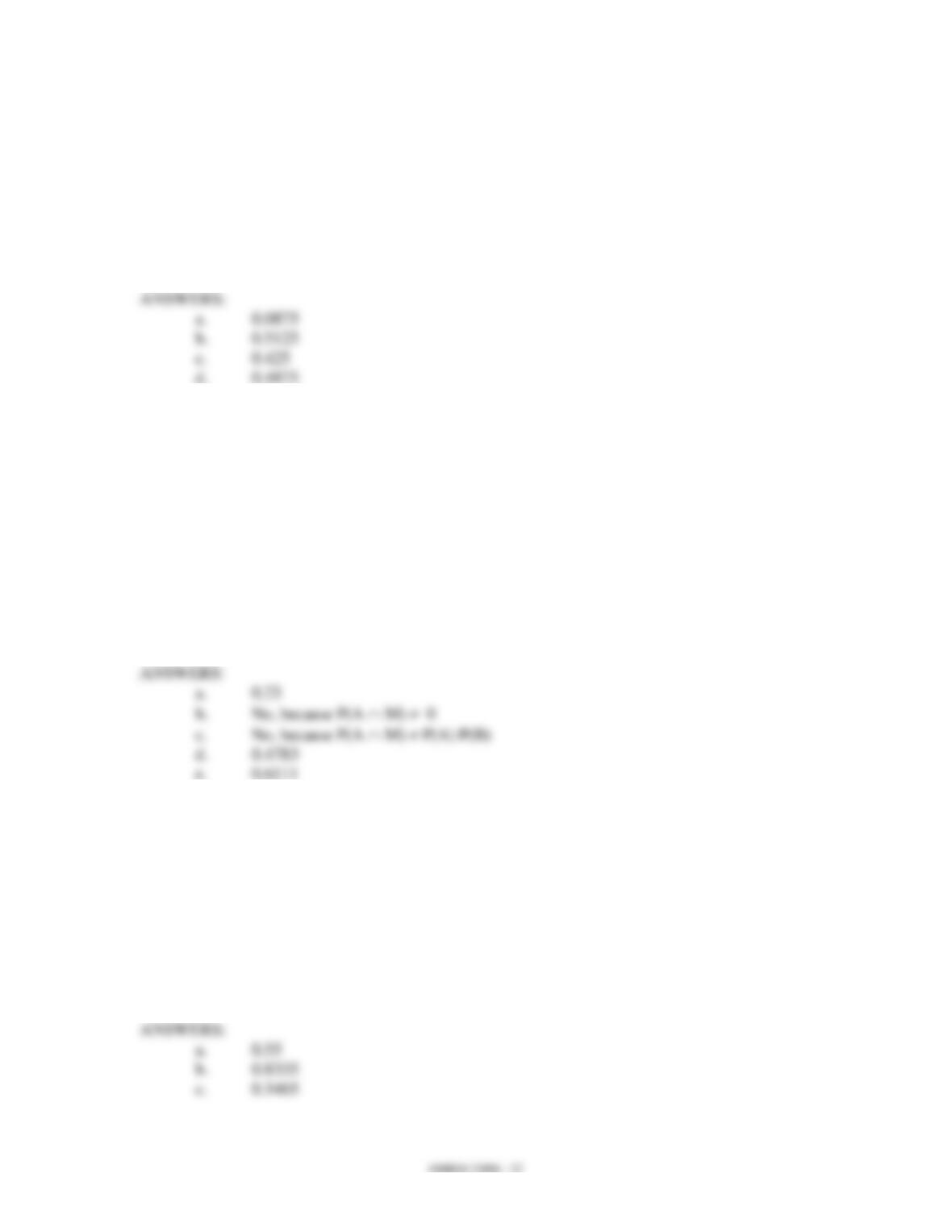as yours) who applied to University A were accepted, while University B accepted 35%
of the applicants. Assume events are independent of each other.
a. What is the probability that you will be accepted in both universities?
b. What is the probability that you will be accepted to at least one graduate
program?
c. What is the probability that one and only one of the universities will accept you?
d. What is the probability that neither university will accept you?
25. Assume you have applied for two scholarships, a Merit scholarship (M) and an Athletic
scholarship (A). The probability that you receive an Athletic scholarship is 0.18. The
probability of receiving both scholarships is 0.11. The probability of getting at least one of
the scholarships is 0.3.
a. What is the probability that you will receive a Merit scholarship?
b. Are events A and M mutually exclusive? Why or why not? Explain.
c. Are the two events, A and M, independent? Explain, using probabilities.
d. What is the probability of receiving the Athletic scholarship given that you have
been awarded the Merit scholarship?
e. What is the probability of receiving the Merit scholarship given that you have
been awarded the Athletic scholarship?
26. In the two upcoming basketball games, the probability that UTC will defeat Marshall is
0.63, and the probability that UTC will defeat Furman is 0.55. The probability that UTC
will defeat both opponents is 0.3465.
a. What is the probability that UTC will defeat Furman given that they defeat
Marshall?
b. What is the probability that UTC will win at least one of the games?
c. What is the probability of UTC winning both games?
d. Are the outcomes of the games independent? Explain and substantiate your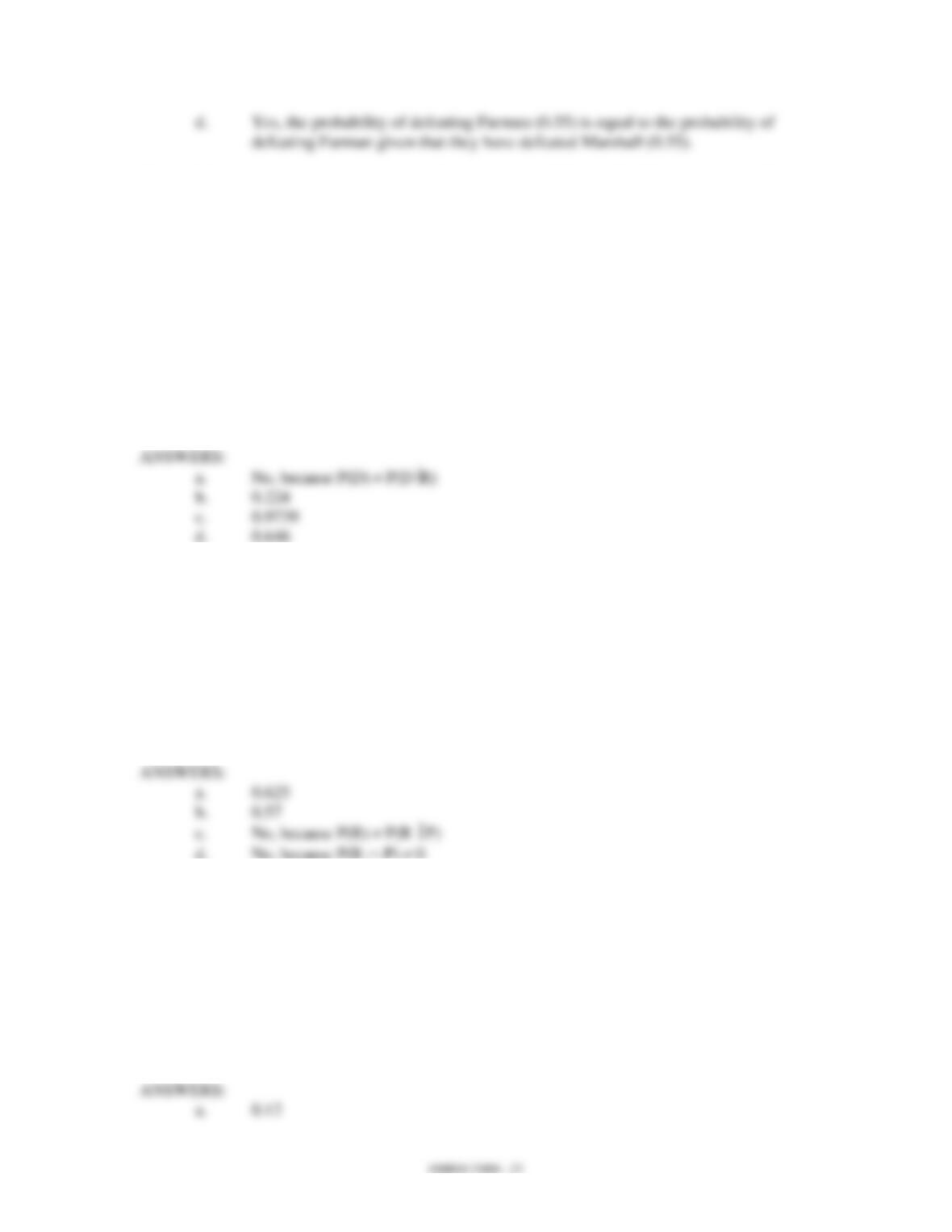27. The probability of an economic decline in the year 2001 is 0.23. There is a probability of
0.64 that we will elect a republican president in the year 2000. If we elect a republican
president, there is a 0.35 probability of an economic decline. Let “D” represent the event
of an economic decline, and “R” represent the event of election of a Republican
president.
a. Are “R” and “D” independent events?
b. What is the probability of electing a Republican president in 2000 and an
economic decline in the year 2001?
c. If we experience an economic decline in the year 2001, what is the probability
that a Republican president will have been elected in the year 2000?
d. What is the probability of economic decline in 2001 or a Republican president
elected in the year 2000 or both?
28. As a company manager for Claimstat Corporation there is a 0.40 probability that you will
be promoted this year. There is a 0.72 probability that you will get a promotion or a
raise. The probability of getting a promotion and a raise is 0.25.
a. If you get a promotion, what is the probability that you will also get a raise?
b. What is the probability of getting a raise?
c. Are getting a raise and being promoted independent events? Explain using
probabilities.
d. Are these two events mutually exclusive? Explain using probabilities.
29. An applicant has applied for positions at Company A and Company B. The probability
of getting an offer from Company A is 0.4, and the probability of getting an offer from
Company B is 0.3. Assuming that the two job offers are independent of each other, what
is the probability that
a. the applicant gets an offer from both companies?
b. the applicant will get at least one offer?
c. the applicant will not be given an offer from either company?
d. Company A does not offer the applicant a job, but Company B does?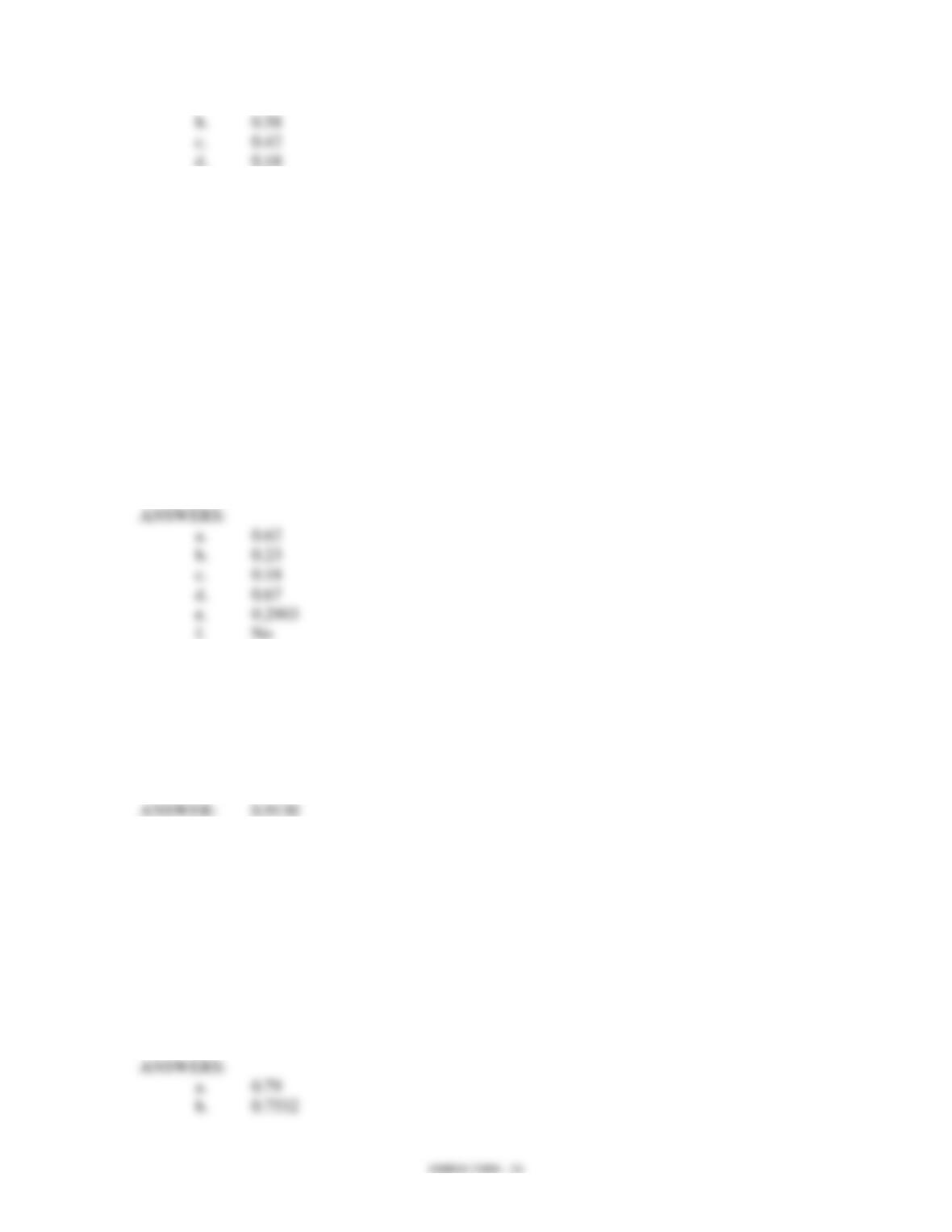30. A corporation has 15,000 employees. Sixty-two percent of the employees are male.
Twenty-three percent of the employees earn more than \$30,000 a year. Eighteen percent
of the employees are male and earn more than \$30,000 a year.
a. If an employee is taken at random, what is the probability that the employee is
male?
b. If an employee is taken at random, what is the probability that the employee
earns more than \$30,000 a year?
c. If an employee is taken at random, what is the probability that the employee is
male and earns more than \$30,000 a year?
d. If an employee is taken at random, what is the probability that the employee is
male or earns more than \$30,000 a year or both?
e. The employee taken at random turns out to be male. Compute the probability
that he earns more than \$30,000 a year.
f. Are being male and earning more than \$30,000 a year independent?
31. A statistics professor has noted from past experience that a student who follows a
program of studying two hours for each hour in class has a probability of 0.9 of getting a
grade of C or better, while a student who does not follow a regular study program has a
probability of 0.2 of getting a C or better. It is known that 70% of the students follow the
study program. Find the probability that if a student who has earned a C or better grade,
he/she followed the program.
(GMAT) indicated that students who have spent at least five hours studying GMAT
review guides have a probability of 0.85 of scoring above 400. Students who do not
spend at least five hours reviewing have a probability of 0.65 of scoring above 400. It
has been determined that 70% of the business students spent at least five hours reviewing
for the test.
a. Find the probability of scoring above 400.
b. Find the probability that given a student scored above 400, he/she spent at least
five hours reviewing for the test.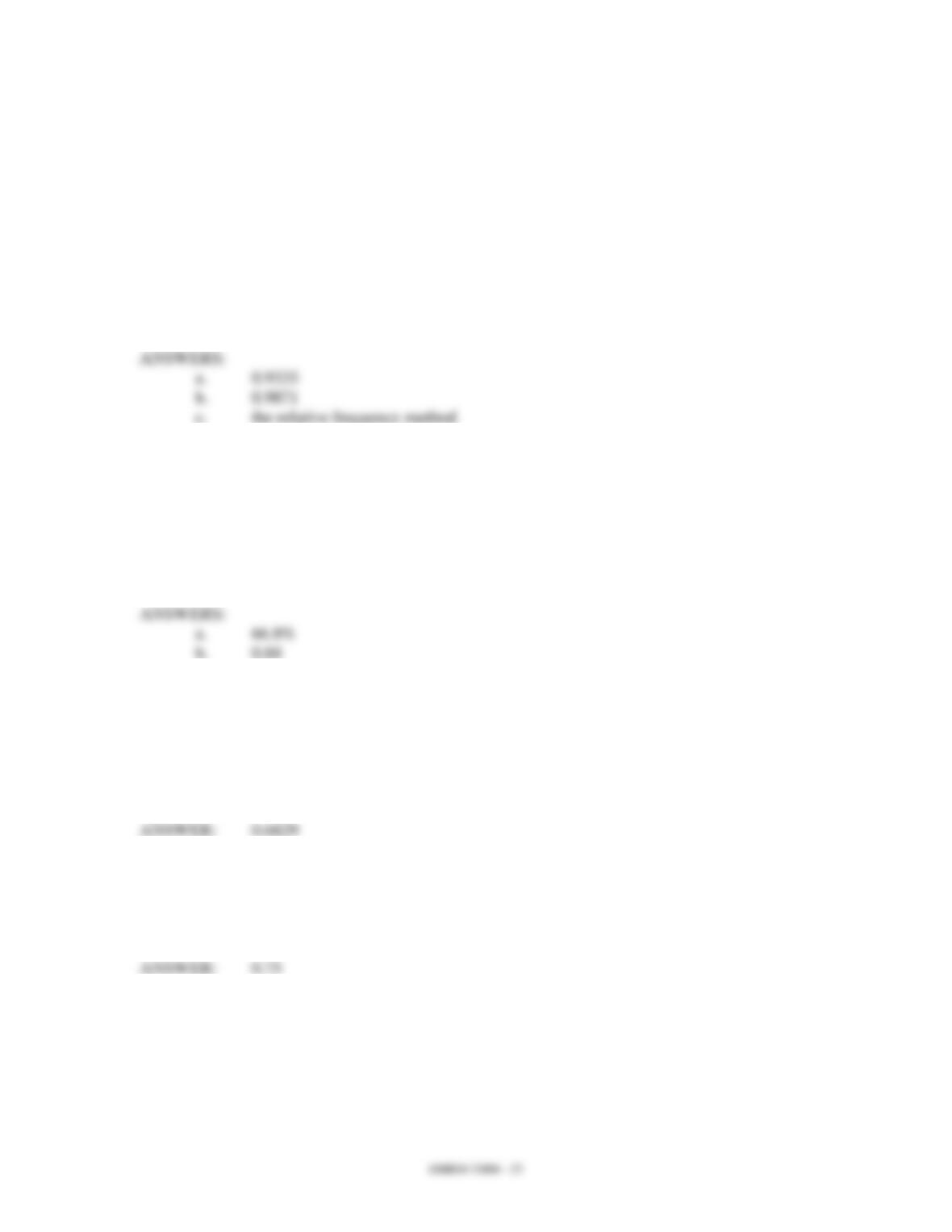33. A machine is used in a production process. From past data, it is known that 97% of the
time the machine is set up correctly. Furthermore, it is known that if the machine is set
up correctly, it produces 95% acceptable (non-defective) items. However, when it is set
up incorrectly, it produces only 40% acceptable items.
a. An item from the production line is selected. What is the probability that the
selected item is non-defective?
b. Given that the selected item is non-defective, what is the probability that the
machine is set up correctly?
c. What method of assigning probabilities was used here?
34. In a recent survey in a Statistics class, it was determined that only 60% of the students
attend class on Fridays. From past data it was noted that 98% of those who went to class
on Fridays pass the course, while only 20% of those who did not go to class on Fridays
passed the course.
a. What percentage of students is expected to pass the course?
b. Given that a person passes the course, what is the probability that he/she attended
classes on Fridays?
35. Thirty-five percent of the students who enroll in a statistics course go to the statistics
laboratory on a regular basis. Past data indicates that 40% of those students who use the
lab on a regular basis make a grade of B or better. On the other hand, 10% of students
who do not go to the lab on a regular basis make a grade of B or better. If a particular
student made an A, determine the probability that she or he used the lab on a regular
basis.
36. In a city, 60% of the residents live in houses and 40% of the residents live in apartments.
Of the people who live in houses, 20% own their own business. Of the people who live
in apartments, 10% own their own business. If a person owns his or her own business,
find the probability that he or she lives in a house.
37. A market study taken at a local sporting goods store showed that of 20 people questioned,
6 owned tents, 10 owned sleeping bags, 8 owned camping stoves, 4 owned both tents and
camping stoves, and 4 owned both sleeping bags and camping stoves. Let Event A =
owns a tent, Event B = owns a sleeping bag, Event C = owns a camping stove,
and Sample Space = 20 people questioned.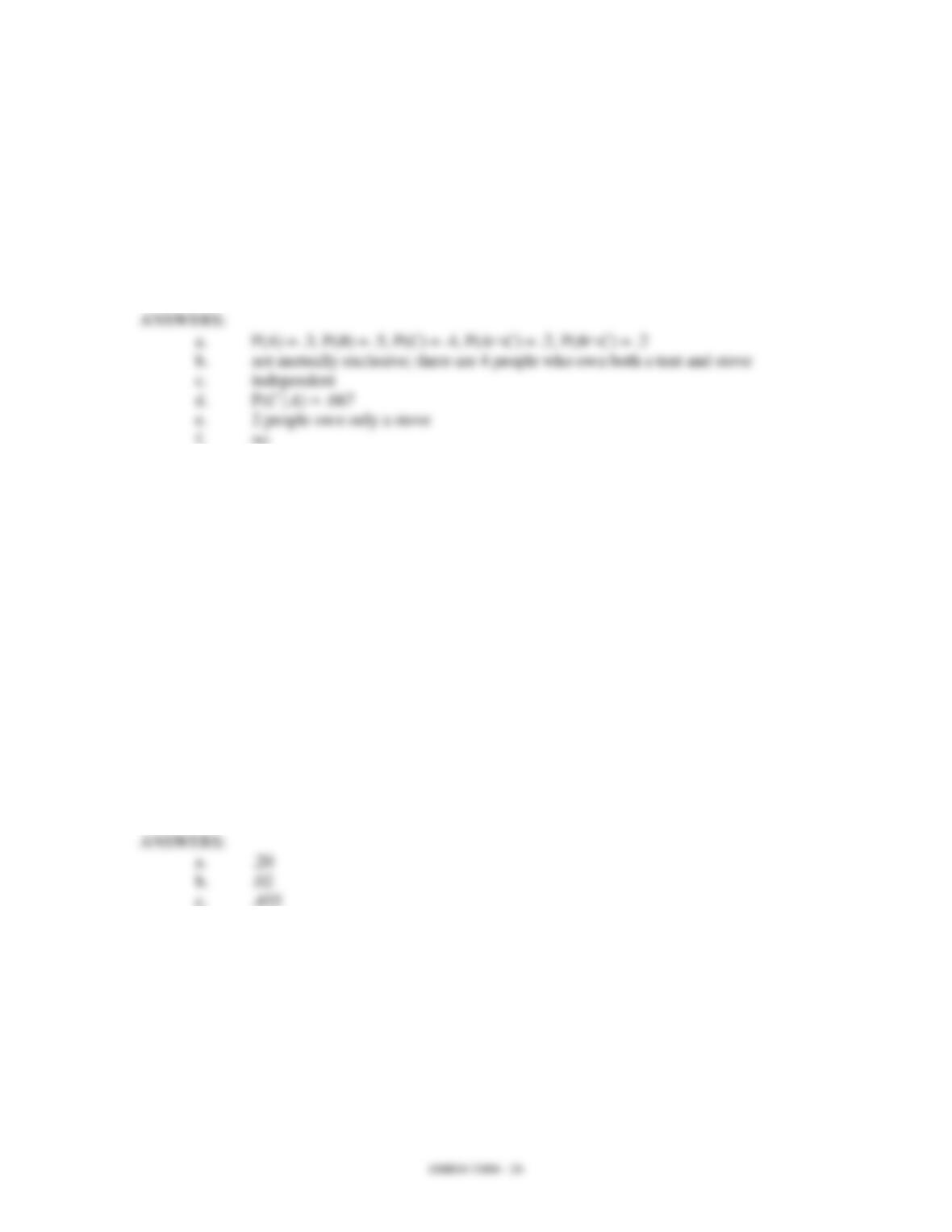a. Find P(A), P(B), P(C), P(AC), P(BC).
b. Are the events A and C mutually exclusive? Explain briefly.
c. Are the events B and C independent events? Explain briefly.
d. If a person questioned owns a tent, what is the probability he also owns a
camping stove?
e. If two people questioned own a tent, a sleeping bag, and a camping stove, how
many own only a camping stove?
f. Is it possible for 3 people to own both a tent and a sleeping bag, but not a
camping stove?
38. The Board of Directors of Bidwell Valve Company has made the following estimates for
the upcoming year's annual earnings:
P(earnings lower than this year) = .30
P(earnings about the same as this year) = .50
P(earnings higher than this year) = .20
After talking with union leaders, the human resource department has drawn the following
conclusions:
P(Union will request wage increase | lower earnings next year) = .25
P(Union will request wage increase | same earnings next year) = .40
P(Union will request wage increase | higher earnings next year) = .90
a. Calculate the probability that the company earns the same as this year and the
union requests a wage increase.
b. Calculate the probability that the company has higher earnings next year and the
union does not request a wage increase.
c. Calculate the probability that the union requests a wage increase.
39. An accounting firm has noticed that of the companies it audits, 85% show no inventory
shortages, 10% show small inventory shortages and 5% show large inventory shortages.
The firm has devised a new accounting test for which it believes the following
probabilities hold:
P(company will pass test | no shortage) = .90
P(company will pass test | small shortage)
= .50
P(company will pass test | large shortage) = .20
a. If a company being audited fails this test, what is the probability of a large or
small inventory shortage?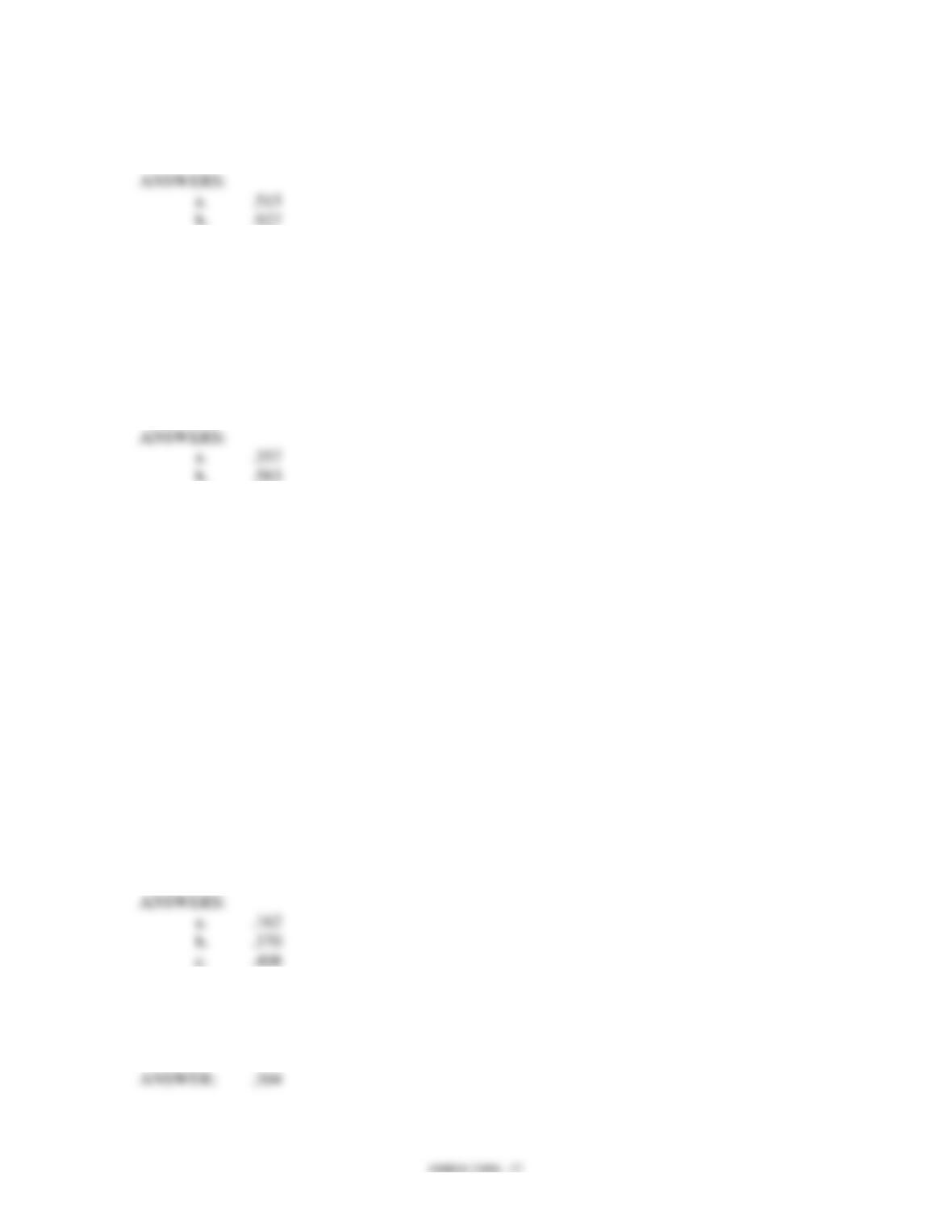b. If a company being audited passes this test, what is the probability of no
inventory shortage?
40. Global Airlines operates two types of jet planes: jumbo and ordinary. On jumbo jets,
25% of the passengers are on business while on ordinary jets 30% of the passengers are
on business. Of Global's air fleet, 40% of its capacity is provided on jumbo jets. (Hint:
you have been given two conditional probabilities.)
a. What is the probability a randomly chosen business customer flying with Global
is on a jumbo jet?
b. What is the probability a randomly chosen non-business customer flying with
Global is on an ordinary jet?
41. Safety Insurance Company has compiled the following statistics. For any one-year
period:
P(accident | male driver under 25) = .22
P(accident | male driver over 25) = .15
P(accident | female driver under 25) = .16
P(accident | female driver over 25) = .14
The percentage of Safety's policyholders in each category is:
Male Under 25 20%
Male Over 25 40%
Female Under 25 10%
Female Over 25 30%
a. What is the probability that a randomly selected policyholder will have an
accident within the next year?
b. Given that a driver has an accident, what is the probability the driver is a male
over 25?
c. Given that a driver has no accident, what is the probability the driver is a female?
42. Super Cola sales breakdown as 80% regular soda and 20% diet soda. Men purchase 60%
of the regular soda, but only 30% of the diet soda. If a woman purchases Super Cola,
what is the probability that it is a diet soda?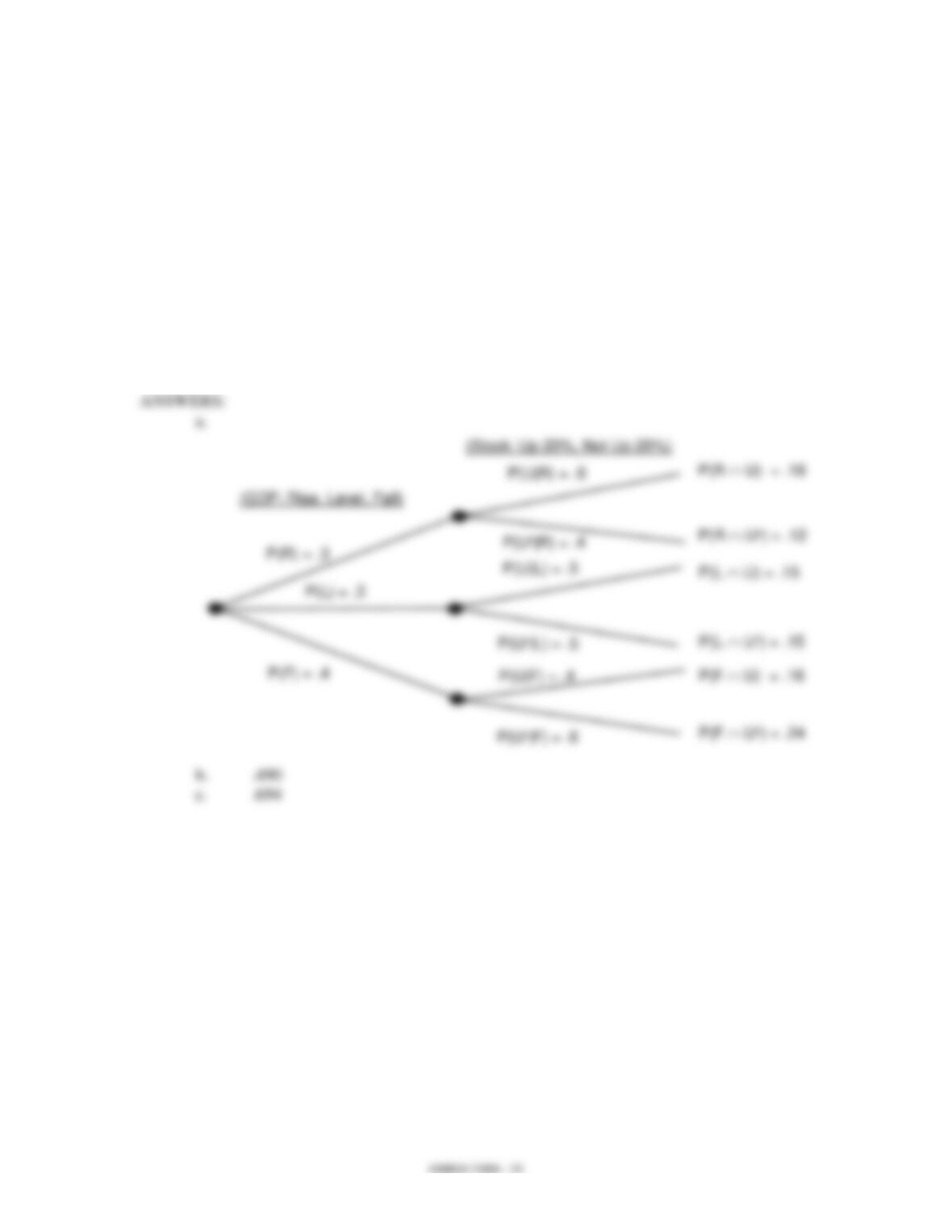43. An investment advisor recommends the purchase of shares in Infogenics, Inc. He has
P(Stock goes up 20% | Rise in GDP) = .6
P(Stock goes up 20% | Level GDP) = .5
P(Stock goes up 20% | Fall in GDP) = .4
An economist has predicted that the probability of a rise in the GDP is 30%, whereas the
probability of a fall in the GDP is 40%.
a. Draw a tree diagram to represent this multiple-step experiment.
b. What is the probability that the stock will go up 20%?
c. We have been informed that the stock has gone up 20%. What is the probability
of a rise or fall in the GDP?

## Trusted by Thousands ofStudents

Here are what students say about us.